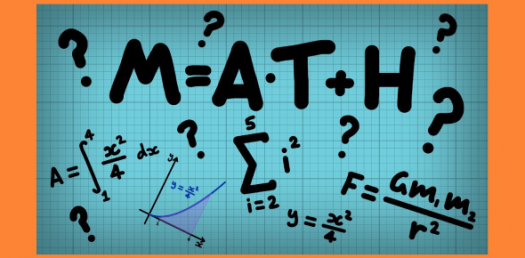# Equation Trivia Quiz: Math Questions!

25 Questions | Total Attempts: 551SettingsWelcome to the equation trivia quiz questions that are specifically designed to see just how good your math skills are. It is made up of different types of math problems that you will need to understand if you wish to pass your exams. Give it a try and get as much practice as you need to get them all right.

• 1.
If x+14=20, what does x equal?
• 2.
If 20x=100, what does x equal?
• 3.
If 2x-5=25, what does x equal?
• 4.
If -2x+31=-5+10x, what does x equal?
• 5.
Solve for t if 23q+t=10
• 6.
If 10|x+2|=50, what is x?
• 7.
If x+20=100, what is x?
• 8.
If 7x=21, what is x?
• 9.
If 5x+5=20, what is x?
• 10.
If -4x+5=x-10, what is x?
• 11.
If 10r-100t=50, what is r?
• 12.
If 10|x-2|=250, what is x?
• 13.
If 7+t=10, what is t?
• 14.
If 3x=36, what is x?
• 15.
If 20x-15=-5x+10, what is x?
• 16.
If 11x+11t=121, what is x?
• 17.
If 2|4x+2|=20, what is x?
• 18.
If x-2=27, what is x?
• 19.
If 2y-2=44, what is y?
• 20.
If 5x+10=50, what is x?
• 21.
If 11x=121, what is x?
• 22.
If t+55x=100, what is t equal to?
• 23.
If 2x-30=20, what is x?
• 24.
If 50x=100, what is x?
• 25.
If 3|x+6|=36, what is x?
Related Topics